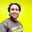Related Tags

machine learning
community creator

# Machine Learning terminologiesTAPAS ADHIKARY

If you are a newbie to Machine Learning(ML), you may find some of the terminologies a bit overwhelming. In order to understand what these terms mean, you need to understand their high-level meaning.

Field Type
Model A representation of what a Machine Learning system has learned from the training.
Training The process of building a Machine Learning model. Training is comprised of various examples to help to build the model.
Examples One row of a dataset helps in training a model. An example consists of the input data and a Label.
Feature A feature is an input variable that helps with the Prediction.
Label In Supervised Learning, Label is the possible result of an example.
Dataset A dataset is a collection of examples.
Prediction Prediction is the output of a model based on the input examples.
Classification Model A model that helps differentiate between two or more discrete models (e.g., spam and non-spam emails).
Supervised Learning Supervised machine learning is about Training a model using the input data and respective Label.
Image Classification A process that classifies objects and patterns in an image.
Unsupervised Learning Unsupervised machine learning is about Training a model to find patterns in an unlabeled dataset.
Clustering In Unsupervised machine learning, related examples are grouped together in a process called Clustering.
Regression Model While a classification model outputs discrete values, a regression model outputs continuous values (e.g., values between 0 to 1).

You can read more about these and other Machine Learning terminologies here.

RELATED TAGS

machine learning
community creator

CONTRIBUTORTAPAS ADHIKARY
RELATED COURSES

View all Courses

Keep Exploring

Learn in-demand tech skills in half the time